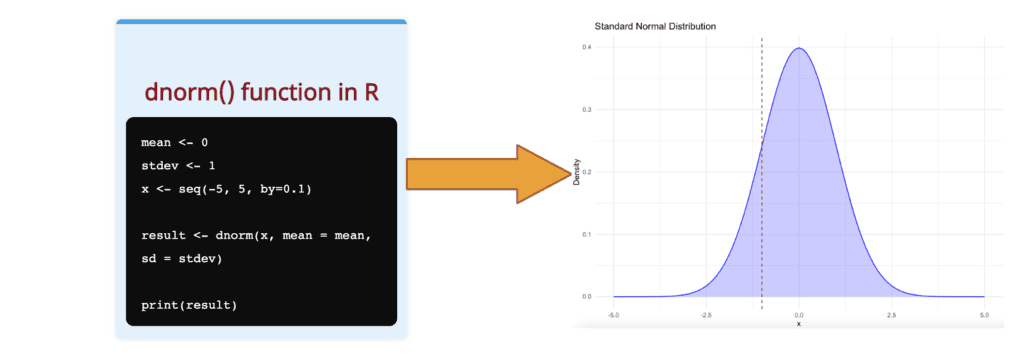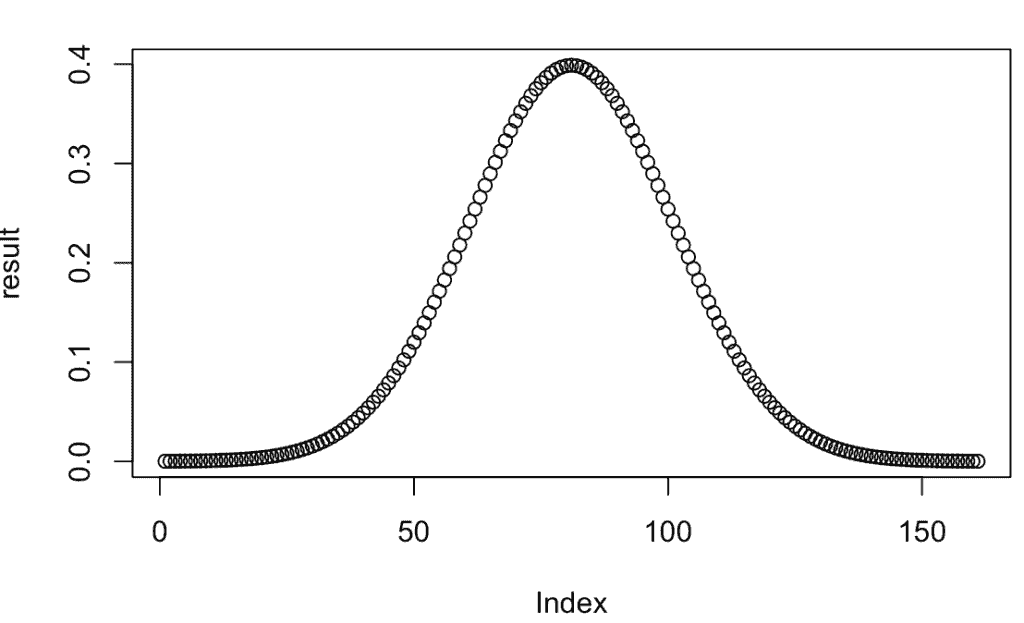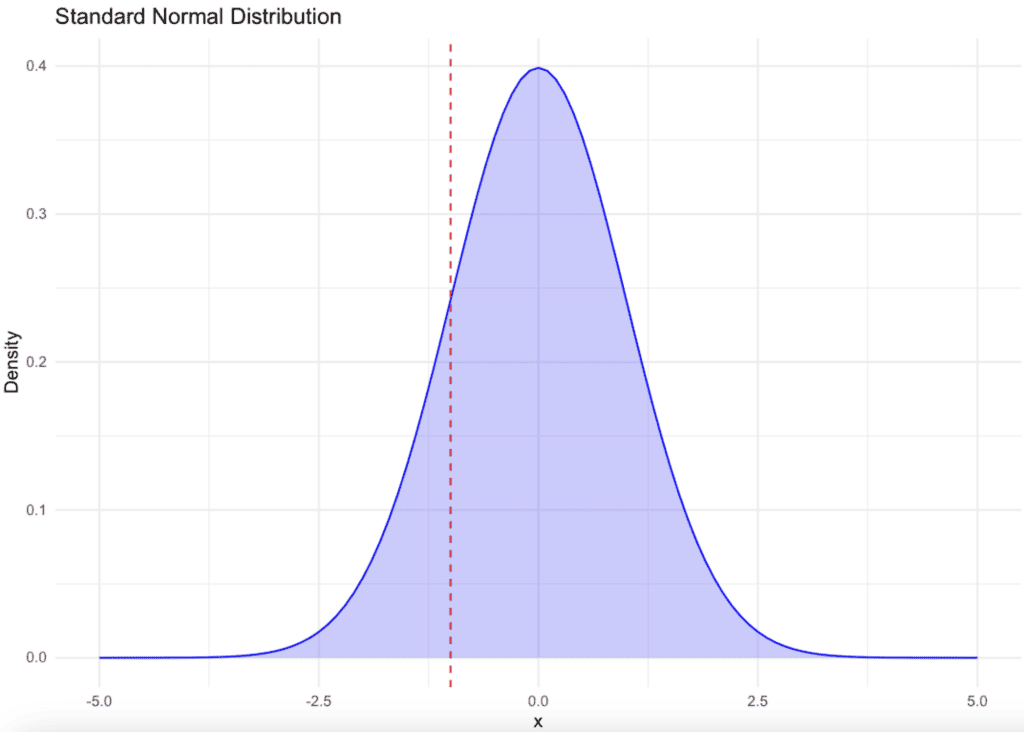# How to Use dnorm() Function in R

The dnorm() function in R calculates the value of the probability density function (pdf) of the normal distribution given a specific random variable x, a population mean μ, and the population standard deviation σ.

## Formula## Syntax

``````dnorm(x, mean, sd)
``````

## Parameters

1. x: It is a numeric vector of values for which the PDF of the normal distribution should be computed.
2. mean: It is the mean of the normal distribution and is set to 0 by default.
3. sd: It is the standard deviation of the normal distribution and is set to 1 by default.

If the mean and sd are not specified, they assume the default values of 0 and 1, respectively.

## Return value

It returns the value of the probability density function (pdf) of the normal distribution.

## Visualization## Example 1: Usage of dnorm() function

Define the mean and standard deviation of the normal distribution and the value(s) at which you want to evaluate the density function. In the next step, the dnorm() function is used to calculate the density at those values.

``````mean <- 0
stdev <- 1
x <- -1

result <- dnorm(x, mean = mean, sd = stdev)

print(result)``````

Output

`` 0.2419707``

## Example 2: Visualization using plot() function

``````seq(-4, 4, by = 0.05)

result <- dnorm(seq(-4, 4, by = 0.05))

plot(result)
``````

Output## Example 3: Visualization using ggplot2

``````library(ggplot2)

# Generate x values
x_values <- seq(-5, 5, by = 0.025)

# Compute y values using the dnorm function
y_values <- dnorm(x_values, mean = 0, sd = 1)

# Point of interest
poi_x <- 0
poi_y <- dnorm(poi_x, mean = 0, sd = 1)

# Plotting
df <- data.frame(x = x_values, y = y_values)
ggplot(df, aes(x, y)) +
geom_line(color = "blue") +
geom_point(aes(x = poi_x, y = poi_y), color = "red", size = 4) +
labs(
title = "Density of Standard Normal Distribution at x=0",
x = "x", y = "Density"
) +
theme_minimal() +
theme(legend.position = "none")``````You can see the plot of the standard normal distribution. The curve is blue, with a red point at x=0 suggesting the density at that specific point.

## Example 4: Calculating probability density for a range of values

You can calculate the probability density for a range of values using the dnorm() function in combination with the sapply() function.

``````x <- c(-3, -2, -1, 0, 1, 2, 3)
mean <- 0
sd <- 1
log <- FALSE

sapply(x, dnorm, mean=mean, sd=sd, log=log)``````

Output

`````` 0.004431848 0.053990967 0.241970725 0.398942280 0.241970725 0.053990967
 0.004431848``````

## Example 5: Pass mean = 0 and sd = 1

``````dt <- dnorm(0, mean = 0, sd = 1)
dt``````

Output

`` 0.3989423``

## Example 6: dnorm(0)

``````dt <- dnorm(0)
dt``````

Output

`` 0.3989423``

rnorm() function

pnorm() function

qnorm() function